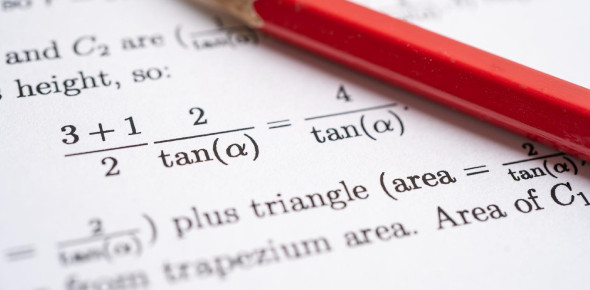# Algebra Review Practice Test

10 Questions | Total Attempts: 346SettingsTest your knowledge of algebra with this algebra review practice test and assess yourself whether you still need some more practice or you've become an expert. If you're looking for the best algebra quiz questions with answers, look nowhere. Simply play the quiz below and improve your basics. Here, you'll be asked questions related to the foil method too. Do you know what's that? Why don't you take up this test and check your memory? All the best, dear!

• 1.
Classify as a mononomial, binomial, or trinomial 3x^2
• 2.
Find the degree 8xy^2 - 2x + 1xy^3
• 3.
Evaluate x^3 + 2x - 4 when x=-2
• 4.
Subtract: (7x^2 + 2x-5) - (3x^2 -2x-1). Options: A. 4x^2+4x-6 B. 4x^2-6, C. 4x+4x-6 D. 4x^3+4x-6
• 5.
What does FOIL stand for?
• 6.
Hint foil method: (2x+3)(x-2) Options: A. 2x^2-x-6 B. 2x-x-6 C.x^2-x-6 D. None
• 7.
(3x-3)(2x+2) Options: A. 6x2-6x-6 B. 6x2-6x C. 6x2+6x-6 D. None
• 8.
3x-13x^2+14x-2 Options: A. -13x+17x-2 B. -13x^2+17x-2 C. -13x^2-17x-2 D. None
• 9.
2y^2(y^2 + 5y) Options: A. 2y^4-10y^3 B. 2y^4+10y^2 C. 2y^4+10y^3 D. None
• 10.
3xy-21x divided by 24xy
Related TopicsBack to top